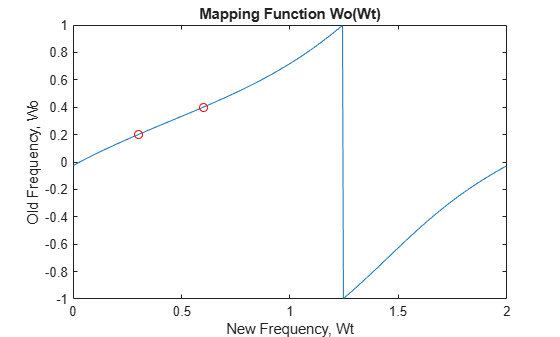# allpassbpc2bpc

Allpass filter for complex bandpass transformation

## Syntax

``[AllpassNum,AllpassDen] = allpassbpc2bpc(Wo,Wt)``

## Description

````[AllpassNum,AllpassDen] = allpassbpc2bpc(Wo,Wt)` returns the numerator, `AllpassNum`, and the denominator, `AllpassDen`, of the first-order allpass mapping filter for performing a complex bandpass to complex bandpass frequency transformation. For more information, see Complex Bandpass to Complex Bandpass Frequency Transformation.```

## Examples

collapse all

This example shows how to design allpass mapping filter, changing the complex bandpass filter with the band edges at ${W}_{o1}=0.2$ and ${W}_{o2}=0.4$ to the new band edges of ${W}_{t1}=0.3$ and ${W}_{t2}=0.6$. Find the frequency response of the allpass mapping filter:

```Wo = [0.2, 0.4]; Wt = [0.3, 0.6]; [AllpassNum, AllpassDen] = allpassbpc2bpc(Wo, Wt); [ha, f] = freqz(AllpassNum, AllpassDen, 'whole'); plot(f/pi,-angle(ha)/pi, Wt, Wo, 'ro') title('Mapping Function Wo(Wt)'); xlabel('New Frequency, Wt'); ylabel('Old Frequency, Wo');```## Input Arguments

collapse all

Frequency values to be transformed from the prototype filter, specified as a real vector with values in the range `(-1,1)`.

Data Types: `single` | `double`

Desired frequency locations in the transformed target filter, specified as a real vector with values in the range `(-1,1)`.

Data Types: `single` | `double`

## Output Arguments

collapse all

Numerator of the mapping filter, returned as a complex-valued vector.

Data Types: `double`
Complex Number Support: Yes

Denominator of the mapping filter, returned as a complex-valued vector.

Data Types: `double`
Complex Number Support: Yes

collapse all

### Complex Bandpass to Complex Bandpass Frequency Transformation

Complex bandpass to complex bandpass frequency transformation effectively places two features of an original filter, located at frequencies Wo1 and Wo2, at the required target frequency locations Wt1 and Wt2. It is assumed that Wt2 is greater than Wt1. In most of the cases the features selected for the transformation are the band edges of the filter passbands. In general it is possible to select any feature; e.g., the stopband edge, the DC, the deep minimum in the stopband, or other ones.

Frequencies must be normalized to be between -1 and 1, with 1 corresponding to half the sample rate.

Relative positions of other features of an original filter do not change in the target filter. This means that it is possible to select two features of an original filter, F1 and F2, with F1 preceding F2. Feature F1 will still precede F2 after the transformation. However, the distance between F1 and F2 will not be the same before and after the transformation.

This transformation can also be used for transforming other types of filters; e.g., complex notch filters or resonators can be repositioned at two distinct desired frequencies at any place around the unit circle. This is very attractive for adaptive systems.

## Version History

Introduced in R2011a